Courses

# Test: Previous Year Questions: Chemical Kinetics

## 25 Questions MCQ Test Chemistry Class 12 | Test: Previous Year Questions: Chemical Kinetics

Description
This mock test of Test: Previous Year Questions: Chemical Kinetics for JEE helps you for every JEE entrance exam. This contains 25 Multiple Choice Questions for JEE Test: Previous Year Questions: Chemical Kinetics (mcq) to study with solutions a complete question bank. The solved questions answers in this Test: Previous Year Questions: Chemical Kinetics quiz give you a good mix of easy questions and tough questions. JEE students definitely take this Test: Previous Year Questions: Chemical Kinetics exercise for a better result in the exam. You can find other Test: Previous Year Questions: Chemical Kinetics extra questions, long questions & short questions for JEE on EduRev as well by searching above.
QUESTION: 1

### In a zero-order reaction for every 10° rise of temperature, the rate is doubled. If the temperature is increased from 10°C to 100°C, the rate of the reaction will become

Solution:

For 10 degree rise in temperature, n = 1

so rate = 2n = 21 = 2

When temperature is increased from 10 deg C to 100 deg C change in temperature = 100-10 = 90 deg C i.e n=9

so, rate = 29 = 512 times.

QUESTION: 2

### A chemical reaction [2A] + [2B] + [C] → product follows the rate equation :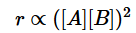then order of reaction is -            [AIEEE-2002]

Solution:

The correct answer is option C
3A ➡ 2B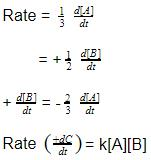Thus, the order of reaction with respect to A = 1
The order of reaction with respect to B =1
Total order of reaction = 1+1 = 2

QUESTION: 3

### The unit of rate constant of first & second order reaction is respectively -

Solution:

The correct answer is option A
The unit of the rate constant K is (Molarity)1−n time−1.
Here, n is the order of the reaction.
For first order reaction, n=1.
The unit of the rate constant K is (Molarity)1−1sec−1 = sec−1.
For zero order reaction, n=0.
The unit of the rate constant K is (Molarity)1−0sec−1 =(Molarity)sec−1 = (M) sec−1.

QUESTION: 4

In the equation Kt = log C0 – log Ct, the curve between t and log Ct is -

[AIEEE-2002]

Solution:

The correct answer is option A
Compare straight line equation
(y = mx + c) with Kt = -log (Ct) + log(C0)
where,
K being constant,
"t" is equivalent to "y" in straight line equation
(-1) is slope for equation which is "m" in straight line equation
log (Ct) is x-component
& log (C0) is y-intercept.

QUESTION: 5

Consider following two reactions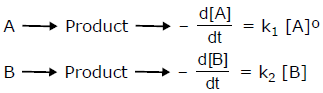Units of k1 and k2 are expressed in terms of molarity (mol L–1) and time (sec–1) as –

[AIEEE-2002]

Solution:

The correct answer is Option C.
Unit of rate of reaction =sec−1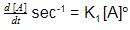∴ Units of K1 = sec−1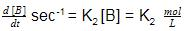∴ Unit of K2 = M−1sec−1

QUESTION: 6

H2 gas is adsorbed on the metal surface like tungsten. This follows........ order reaction –

[AIEEE-2002]

Solution:

In simpler words, after a certain period of time , Hydrogen is completely adsorbed on tungsten metal such that its surface becomes completely concentrated with Hydrogen thus the reaction becomes independent of concentration. a rxn independent of concentration is 0 order.

QUESTION: 7

In respect of the equation k = Ae –Ea/RT in chemical kinetics, which one of the following statement is
correct?

[AIEEE-2003]

Solution:

The correct answer is option A
In the Arrhenius Equation,
R is the gas constant.
k is the rate constant.
A is the frequency factor which is also known as Arrhenius factor.
Ea is the activation energy and it is the minimum amount of energy required for any reaction to proceed.
T is for temperature.

QUESTION: 8

For the reaction system: 2NO(g) + O2(g) → 2NO2(g) volume is suddenly reduced to half its value by increasing the pressure on it. If the reaction is of first order with respect to O2 and second order with repect to NO, the rate of reaction will –

[AIEEE-2003]

Solution:

The correct answer is option A
rate=k[NO]2[O2]
As, C = n/V
On making the volume half, concentration doubles and rate of reaction becomes eight times.

QUESTION: 9

The rate law for a reaction between substances A and B is given by
Rate = k [A]n [B]m

On doubling the concentration of A and halving the concentration of B, the ratio of the new rate to the earlier rate of the reaction will be as–

[AIEEE-2003]

Solution:

The correct answer is Option B.

Rate =k[A]n[B]m

When
A'= 2A and
B'= 0.5B

The new rate becomes Rate'
=[2A]n[0.5]m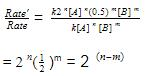QUESTION: 10

In a first order reaction, the concentration of the reactant, decreases from 0.8 M to 0.4 M in 15 minutes. The time taken for the concentration to change from 0.1 M to 0.025 M is -

[AIEEE-2004]

Solution:

The correct answer is  Option A.
The concentration of the reactant decreases from 0.8M to 0.4M in 15 minutes, i.e. t1/2 = 15 minutes. Therefore, the concentration of the reactant will fall from 0.1M to 0.025M in two half lives. i.e.    2t1/2 = 2 × 15 = 30 minutes.

QUESTION: 11

The rate equation for the reaction 2 A + B → C is found to be : rate = k [A] [B] . The correct statement in relation to this reaction is that the

[AIEEE-2004]

Solution:

The correct answer is Option D.

From the rate equation rate =k[A][B], we can say that the reaction is first order in A and first order in B. Hence, the overall order of the reaction is 2.
(A)The unit of k must be mol-1s-1
Hence, the statement A is incorrect.
(B) The half life period is not constant. It is inversely proportional to the concentration of reactants. Hence, the statement B is incorrect.
(C) The rate of formation of C is one half the rate of disappearance of A.
d[C] / dt = −d[A] / 2dt
Hence, the statement C is incorrect.
(D) The value of k is independent of the initial concentration of A and B. Hence, the statement D is correct.

QUESTION: 12

Consider an endothermic reaction X → Y with the activation energies Eb and Ef for the backward and forward reactions, respectively. In general

[AIEEE-2005]

Solution:

The correct answer is option B
Here, Ef ​> Eb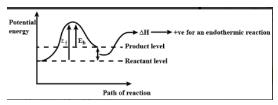QUESTION: 13

A reaction involing two different reactants can never be –

[AIEEE-2005]

Solution:
QUESTION: 14

t1/4 can be taken as the time taken for the concentration of a reactant to drop to 3/4 of its initial value. If the rate constant for a first order reaction is K, t1/4  can be written as –

[AIEEE-2005]

Solution:

The correct answer is option A
Let initial concentration be ao​
After concentration decreases by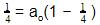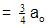Using first order kinetic equation,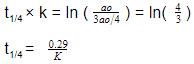QUESTION: 15

A reaction was found to be second order with respect to the concentration of carbon monoxide. If the concentration of carbon monoxide is doubled, with everything else kept the same, the rate of reaction will -

[AIEEE 2006]

Solution:

The correct answer is Option B.
The rate law expression is R=k[CO]2.
When concentration of CO is doubled, the rate law expression becomes ;
R′=k[2CO]2
=4k[CO]2
=4R.

QUESTION: 16

Rate of reaction can be expressed by Arrhenius equation as k = Ae–E/RT , In this equation, E represents

[AIEEE 2006]

Solution:

The correct answer is Option A.

E represents the energy of activation which implies it is the energy below which colliding molecules will not react Arrhenius equation gives the dependence of the rate constant k of a chemical reaction on the absolute temperature T (in Kelvin), where A is the pre-exponential factor (or simply the prefactor), Ea is the activation energy, and R is the Universal gas constant:
By Arrhenius equation, k=Ae-Ea/RT

QUESTION: 17

The following mechanism has been proposed for the reaction of NO with Br2 to form NOBr :
NO(g) + Br2 (g)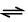NOBr2 (g)
NOBr2 (g) + NO (g) → 2 NOBr (g)
If the second step is the rate determining step, the order of the reaction with respect to NO (g) is -

[AIEEE 2006]

Solution:

The correct answer is option c
NO(g)+Br2(g)     ⇌NOBr2(g)
NOBr2(g)+NO(g) 2NOBr(g)[rate determining step]
Rate of the reaction (r)= K[NOBr2][NO]
Where [NOBR2]=Kc[NO][Br2]
r=K.Kc.[NO][Br2][NO]
r=K’[NO]2[Br2].
The order of the reaction with respect to
NO(g)=2.

QUESTION: 18

The energies of activation for forward and reverse reactions for A2 + B22AB are 180 kJ mol–1 and 200 kJ mol–1 respectively. The presence of a catalyst lowers the activation energy of both (forward and reverse) reactions by 100 kJ mol–1. The enthalpy change of the reaction (A2 + B2 → 2AB) in the presence of catalyst will be (in kJ mol–1) –

[AIEEE 2007]

Solution:

The correct answer is Option D.

In presence of catalyst,
E(forward) = 80kJ/mol  and,
E(reverse) = 100kJ/mol.

Therefore, H(reaction) =E(reverse)−E(forward)
=100−80
=20kJ/mol.

QUESTION: 19

Consider the reaction, 2A + B → Products. When concentration of B alone was doubled, the half-life of B did not change. When the concentration of A alone was doubled, the rate increased by two times. The unit of rate constant for this reaction is –

[AIEEE 2007]

Solution:

The correct answer is Option A.
For first-order reaction, half-life t1/2 =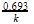Since half-life does not depend on the concentration of reactants.

According to the given data, the order of reaction with respect to B is one, hence, does not change half-life.

Similarly, an order of reaction with respect to A is also one since the rate increases twice on doubling the concentration.
Hence, total order = 1 + 1 = 2

Second order reaction and its unit is Lmol−1s−1.

QUESTION: 20

For a reaction 1/2 A→ 2B, rate of disappearance of ‘A’ related to the rate of appearance of ‘B’ by the expression -

[AIEEE 2008]

Solution:

The correct answer is option A
For the reaction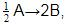rate of disappearance of A is related to rate of appearance of B by the expression: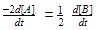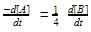QUESTION: 21

The half life period of a first order chemical reaction is 6.93 minutes. The time required for the completion of 99% of the chemical reaction will be (log 2 = 0.301)

[AIEEE 2009]

Solution:

The correct answer is Option B.
The half life period t1/2 = 6.93 min.
The decay constant λ = 0.69/t1/2
=0.693/6.93min
​                  =0.1/min
t = (2.303/λ) log a / a−x
t = 2.303/(0.1/min) log 100/(100-99)
t = 46.06 min
Hence, the time required for 99% completion of the reaction is 46.06 min.

QUESTION: 22

The time for half life period of a certain reaction A → products is 1 hour. When the initial concentration of the reactant ‘A’, is 2.0 mol L–1, how much time does it take for its concentration to come from 0.50 to 0.25 mol L–1 if it is a zero order reaction ?

[AIEEE 2010]

Solution:

The correct answer is Option C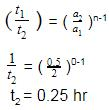QUESTION: 23

The rate of a chemical reaction doubles for every 10ºC rise of temperature. If the temperature is raised by 50ºC, the rate of the reaction increases by about :

[AIEEE 2011]

Solution:

The correct answer is Option B.

For every 10 °C rise of temperature, the rate is doubled. Thus, temperature coefficient of the reaction =2
When temperature is increased by 50°, rate becomes = 2(50/10)
= 25 times
= 32

QUESTION: 24

For a first order (A) → Products, the concentration of A changes from 0.1 M to 0.025 M in 40 minutes. The rate of reaction when the concentration of A is 0.01 M, is :

[AIEEE 2012]

Solution:

k = 2.303/40*log(0.1/0.025)

k = 0.693/20

For a First order reaction,

rate = k[A]

rate = 0.693*10-2/20

The correct option is D.

QUESTION: 25

The rate of a reaction doubles when its temperature changes from 300 K to 310 K. Activation energy of such a reaction will be: (R = 8.314 JK–1 mol–1 and log 2 = 0.301)

[IIT Mains 2013]

Solution:

The correct answer is Option C.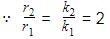Now 2.303log10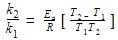2.303log102 =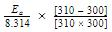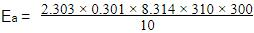=53598.6 J
=53.6 kJmol−1